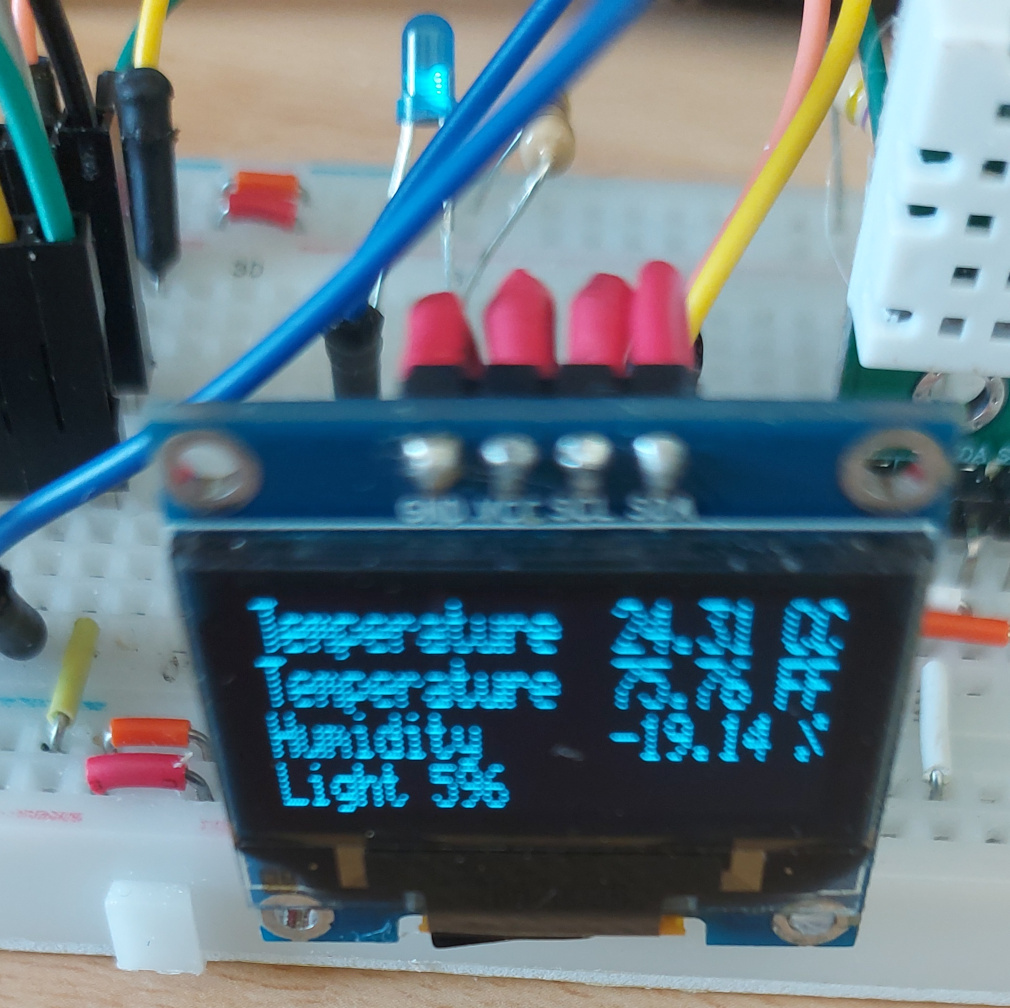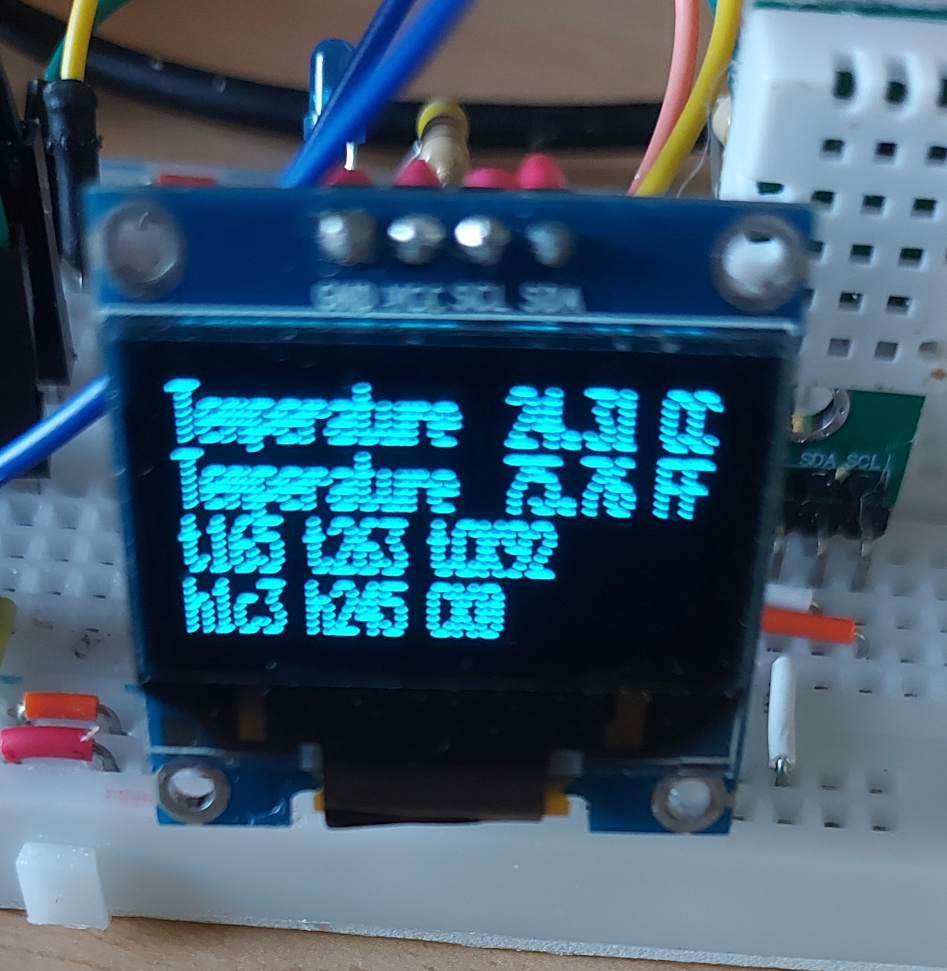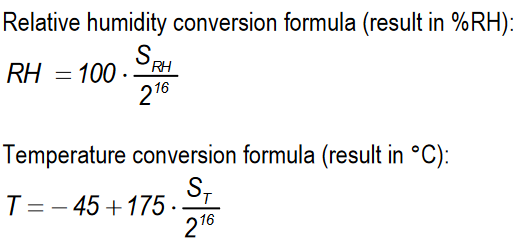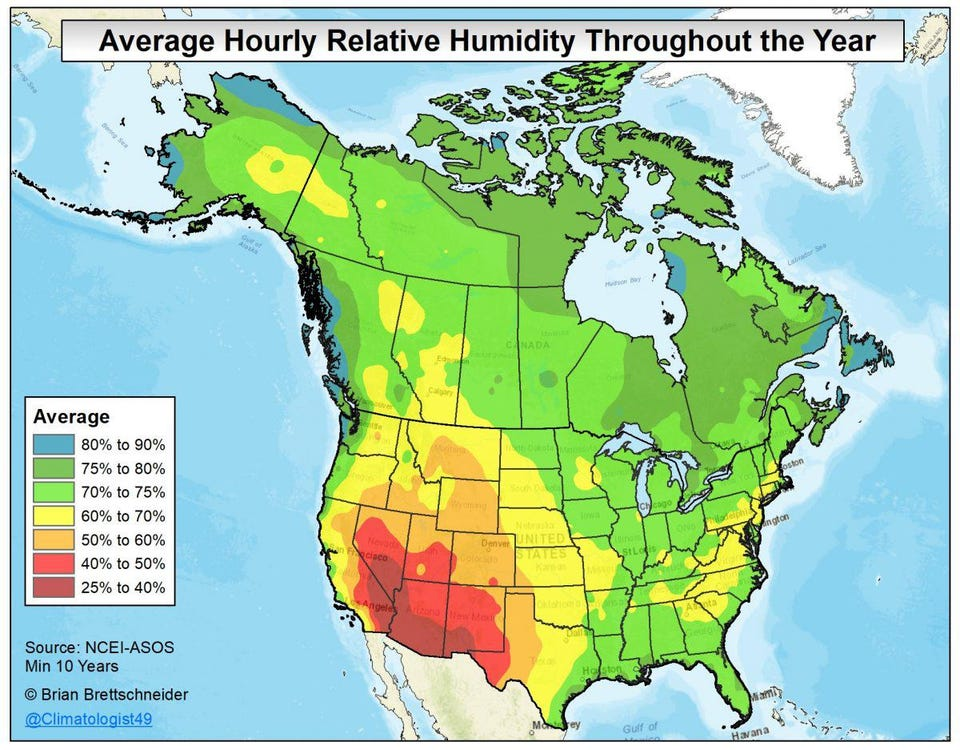# Help needed with Attiny85 and SHTC3 sensor

Calling out to all the great minds here, have little simple programme running on tiny 85
every time I read a sensor I get a correct temperature reading but cannot get a humidity reading at all. I know it’s my lack of knowledge and something I’m doing obviously wrong in trying to retrieve the second block of data from the sensor. I have tried looking at the libraries to see how they achieve the readings but to be perfectly honest looking at some of their code goes completely beyond my simple understanding. The reason I do not wish to use a library is the size restriction. I’m asking for any suggestions or pointers in solving this problem. You might be asking yourself why bother to use a tiny85 in the first place, I just love the simplicity of the tiny, the power usage running on battery and it’s amazing what you can do with just five /6 GPIO pins.
Thank you for looking please find code below ini file at the end.
And here is the link to the datasheet I’ve been trying to use all the sensor

``````#include <Arduino.h>

#include <Arduino.h>
#include <TinyWireM.h>
#include <Tiny4kOLED.h>

#define Led 1
#define Led4 4
#define reset 0x905D
#define sleep_mode 0xB098
#define wake_mode 0x3517
#define measure_mode 0x7CA2
#define measure_mode1 0x5C24

const float FACTOR = 2.2;
//int Light;
float humidity;
float humidityH;
float Temperature;
float TemperatureT;
float fahrenheit;
unsigned int readIndex = 0;          					// the index of the current reading
unsigned int total = 0;              					// the running total
unsigned int Light = 0;             				  // the average

unsigned int readIndexT = 0;                   // the index of the current reading
float totalT = 0;

unsigned int readIndexH = 0;                   // the index of the current reading
float totalH = 0;

void Flash1();
void Measure_mode();
void Flash();
void Get_Light();
void Push_TO_oled();
//################################################################################
void setup() {
//MCUSR &= ~(1<<WDRF); // reset status flag
analogReference(INTERNAL);      // use precise internal reference
//wdt_disable();
//wdt_enable(WDTO_1S);
TinyWireM.begin();

oled.begin();
pinMode(Led,OUTPUT);
pinMode(Led4,OUTPUT);

oled.setFont(FONT6X8);
oled.clear();
oled.switchRenderFrame();
oled.clear();
oled.switchRenderFrame();
oled.on();
oled.println("SHTC3 test");

}

// initialize all the readings to 0:
// }

//############################################################################
void loop() {
//Flash();
//wdt_reset();
oled.setCursor(0,11);
Measure_mode();
Get_Light();
Push_TO_oled();
//Flash1();
delay(10);
}
//##############################################################################
void Measure_mode(){
oled.setCursor(1,0);

TinyWireM.write((int)measure_mode >> 8);
TinyWireM.write((int)measure_mode & 0x00FF);
TinyWireM.endTransmission();

int check1 = TinyWireM.read(); // Do not care. Just a place holder

TinyWireM.endTransmission();
TemperatureT = (temp1 << 8) | (temp2 << 0);

//######################
if (readIndexT >= numReadingsT) {              // if we're at the end of the array...
readIndexT = 0;                              // ...wrap around to the beginning:
}
Temperature = totalT / numReadingsT;           // calculate the average:
//######################
//Convert data to Celsius
Temperature =  -45 + 175 * ((float)Temperature/65535); //2^16 = 65535
fahrenheit = Temperature * (9.0/5) + 32.0;
/*
TinyWireM.write((int)measure_mode1 >> 0);
TinyWireM.write((int)measure_mode1 & 0x00FF);
TinyWireM.endTransmission();
check1 = TinyWireM.read(); // Do not care. Just a place holder
*/
humidity = (hum1 << 8) | (hum2 << 0);
humidity = 100 * (humidity/65535);
totalH = humidity;
//######################
if (readIndexH >= numReadingsH) {              // if we're at the end of the array...
readIndexH = 0;                              // ...wrap around to the beginning:
}
totalH = totalH / numReadingsH;           // calculate the average:
humidityH = totalH;
humidityH=humidityH*10;
//humidityH = 100 * (humidityH/65535);
}
//#################################################################################
void Flash(){
digitalWrite(Led4,HIGH);
delay(50);
digitalWrite(Led4,LOW);
}
//###################################################################################
void Flash1(){
digitalWrite(Led,HIGH);
delay(50);
digitalWrite(Led,LOW);
}
//###################################################################################
void Get_Light(){
if (readIndex >= numReadingsL) {            // if we're at the end of the array...
readIndex = 0;                            // ...wrap around to the beginning:
}
Light = total / numReadingsL;               // calculate the average:
}
//####################################################################################
void Push_TO_oled(){
oled.print(F("Temperature  "));
oled.print(Temperature);
oled.println(F(" C"));
oled.print(F("Temperature  "));
oled.print(fahrenheit);
oled.println(F(" F"));
oled.print(F("Humidity     "));
oled.print(humidity);
oled.println(F(" %"));
oled.print(F("Light "));
oled.print(Light);
oled.print("  ");
oled.print(humidityH);
oled.print("  ");
humidityH=0;
}
//#####################################################################################

[env:attiny]
platform = atmelavr
framework = arduino
board = attiny85
monitor_speed = 115200
lib_deps =
datacute/Tiny4kOLED @ ^2.1.1

I’m not familiar with the sensor you are using, but was it a typo in your code where you are reading hum1 and hum2 because you have commented out the code that reads those two values, but kept the code which uses them in a calculation.

Just wondering.

Cheers,
Norm.

You end the transmission and after that you still read stuff? That works?

Good afternoon gentlemen
it appears to work fine for the temperature but it’s just the humidity that fails dismally I get a reading but it is complete gibberish.sorry about the poor picture. Looking at the data sheet it appears to suggest doing it that way. I certainly bow to your greater knowledge.
Just as a test I’ve tried removing that line and putting it after completing the readings on the sensor and there is still no difference to the humidity reading.

Good afternoon Norman
sorry about the messy code, but I do appear to be reading hum1 & 2 at line 98/99 of the posted code.
Thank you for looking

I’ve tidied up the code a little bit more and reposted it below hoping to make it a bit clearer for anyone looking.

`````` #include <Arduino.h>
#include <TinyWireM.h>
#include <Tiny4kOLED.h>

#define Led 1
#define Led4 4
#define reset 0x905D
#define sleep_mode 0xB098
#define wake_mode 0x3517
#define measure_mode 0x7CA2
#define measure_mode1 0x5C24

const float FACTOR = 2.2;
float humidity;
float Temperature;
float TemperatureT;
float fahrenheit;

unsigned int readIndex = 0;          					// the index of the current reading
unsigned int total = 0;              					// the running total
unsigned int Light = 0;             				  // the average

unsigned int readIndexT = 0;                   // the index of the current reading
float totalT = 0;

void Flash1();
void Measure_mode();
void Flash();
void Get_Light();
void Push_TO_oled();
//################################################################################
void setup() {

analogReference(INTERNAL);      // use precise internal reference

TinyWireM.begin();

oled.begin();
pinMode(Led,OUTPUT);
pinMode(Led4,OUTPUT);

oled.setFont(FONT6X8);
oled.clear();
oled.switchRenderFrame();
oled.clear();
oled.switchRenderFrame();
oled.on();
oled.println("SHTC3 test");
}
//############################################################################
void loop() {
Measure_mode();
Get_Light();
Push_TO_oled();
delay(10);
}
//##############################################################################
void Measure_mode(){
oled.setCursor(1,0);

TinyWireM.write((int)measure_mode >> 8);
TinyWireM.write((int)measure_mode & 0x00FF);
//TinyWireM.endTransmission();

int check1 = TinyWireM.read(); // Do not care. Just a place holder

TinyWireM.endTransmission();
TemperatureT = (temp1 << 8) | (temp2 << 0);

//######################
if (readIndexT >= numReadingsT) {              // if we're at the end of the array...
readIndexT = 0;                              // ...wrap around to the beginning:
}
Temperature = totalT / numReadingsT;           // calculate the average:
//######################
//Convert data to Celsius
Temperature =  -45 + 175 * ((float)Temperature/65535); //2^16 = 65535
fahrenheit = Temperature * (9.0/5) + 32.0;

humidity = (hum1 << 8) | (hum2 << 0);
humidity = 100 * (humidity/65535);

}
//#################################################################################
void Flash(){
digitalWrite(Led4,HIGH);
delay(50);
digitalWrite(Led4,LOW);
}
//###################################################################################
void Flash1(){
digitalWrite(Led,HIGH);
delay(50);
digitalWrite(Led,LOW);
}
//###################################################################################
void Get_Light(){
if (readIndex >= numReadingsL) {            // if we're at the end of the array...
readIndex = 0;                            // ...wrap around to the beginning:
}
Light = total / numReadingsL;               // calculate the average:
}
//####################################################################################
void Push_TO_oled(){
oled.setCursor(0,0);
oled.print(F("Temperature  "));
oled.print(Temperature);
oled.println(F(" C"));
oled.print(F("Temperature  "));
oled.print(fahrenheit);
oled.println(F(" F"));
oled.print(F("Humidity     "));
oled.print(humidity);
oled.println(F(" %"));
oled.print(F("Light "));
oled.print(Light);
oled.print("  ");
}
//#####################################################################################``````

Can you try reading all 6 bytes of sensor data (2 bytes temp, 1 byte temp checksum, 2 bytes humidity, 1 bytes humidity checksum) without ending the transmission early and displaying those hex values (`String asHex = String(some_value, HEX);`) on the OLED? What values do you get?

Sorry Maxgerhardt
just having a problem with the print statement
src\main.cpp:147:20: error: expected primary-expression before ‘asHex’
oled.print(String asHex = String(temp1, HEX));
^~~~~
*** [.pio\build\attiny\src\main.cpp.o] Error 1

``	oled.print(String asHex = String(temp1, HEX));``

The example code was for when you want to assign the result to a variable, you can just use the expression on the right itself to get the converted hex string and pass that as an argument.

``````	oled.print(String(temp1, HEX));
``````

Also print spaces beetwen the values so that we can distinguish them.

sorry about that Hope you can see thistemp1 =165 temp2= 263 check92
hum1=3 hum2=245 check2=0
thank you for being so patient

Sure? The values are hex, it more seems to me like `t1 = 0x64`, `t2 = 0x63`, which would yield as decoded temperature:Since `S_T = 0x6463 = 25699` we would have

T = -45 + 175*25699/65536 = 23.6237

And for relative humidity we’d have with `hum1 = 0xc3`, `hum2 = 0x45`, `hum = 0xc345 = 49989`

RH = 100 * 49989 / 65536 = 76.28

If the previous “Temperature: 24.32 °C” value is from a previous readout, that looks to be correct. Humidity with ~76% RH seems also correct, when I google “average relative humidity” I get answers likeSo at least the read out raw sensor values now seem all correct.

Can you try and use the same readout code (6 bytes, no `endTransmission()` at end) and see what humidity value it spews out?

I have quite a few of these sensors around the house and they are all reading approximately 60 RH.
The value being printed I hope this is in hexadecimal.
And the readout on the display is -32.57

``````	oled.print("t1");
oled.print(String(temp1, HEX));
oled.print(" t2");
oled.print(String(temp2, HEX));
oled.print(" tCK");
oled.println(String(check1, HEX));
oled.print("h1");
oled.print(String(hum1, HEX));
oled.print(" h2");
oled.print(String(hum2, HEX));
oled.print(" CK");
oled.print(String(check2 , HEX));
``````

this might be the problem my understanding of what you mean by all six bites
sorry to be a bit of an idiot

``````	void Measure_mode(){
TinyWireM.write((int)measure_mode >> 8);
TinyWireM.write((int)measure_mode & 0x00FF);
//TinyWireM.endTransmission();

check1 = TinyWireM.read(); // Do not care. Just a place holder

//TinyWireM.endTransmission();

TinyWireM.endTransmission();
TemperatureT = (temp1 << 8) | (temp2 << 0);

//######################
if (readIndexT >= numReadingsT) {              // if we're at the end of the array...
readIndexT = 0;                              // ...wrap around to the beginning:
}
Temperature = totalT / numReadingsT;           // calculate the average:
//######################
//Convert data to Celsius
Temperature =  -45 + 175 * ((float)Temperature/65535); //2^16 = 65535
fahrenheit = Temperature * (9.0/5) + 32.0;

humidity = (hum1 << 8) | (hum2 << 0);
humidity = 100 * (humidity/65535);

}
``````

Here you are requesting only 5 instead of 6 bytes.

Oh I think I now see the problem with this. Since the variable is declared as `int hum1`, aka a signed integer, it has a value range of -32,768 to 32,767 (`int` on an AVR is 16 bits). The result of the OR-ing will also be of type `int`. However, as calculated above, the value of `hum` (pieced together) is 49989. This number is not representable by an `int` since it’s beyond the positive range. What happens is an internal integer-overflow takes place that remaps it to -32,768 + (49,989 - 32,767 - 1), aka something in ~ -15k.

The variable must be an unsigned integer to hold the value correctly (value range 0 to 65535).

So try changing the types of `hum1`, `hum2` to `unsigned int`. It also doesn’t hurt to add `unsigned` casts to the conversion logic, such as

``````  humidity = (float)( (unsigned)( (hum1 << 8u) | (hum2 << 0u) ));
humidity = 100 * (humidity/65535.0f);
``````
1 Like

Maxgerhardt well you manage to do it again all working now. Thank you again ever so much. Do you think I should repost a copy of the working code? in case somebody else’s is mad enough to want to use this sensor without a library.Sure, I think it would be helpful to show the full programWorking code thanks to Maxgerhardt

``````  #include <Arduino.h>
#include <TinyWireM.h>
#include <Tiny4kOLED.h>

#define Led1 1
#define Led4 4
#define reset 0x905D
#define sleep_mode 0xB098
#define wake_mode 0x3517
#define measure_mode 0x7CA2

float humidity;
float humidityH;
float Temperature;
float TemperatureT;
float fahrenheit;

unsigned int readIndex = 0;          					// the index of the current reading
unsigned int total = 0;              					// the running total
unsigned int Light = 0;             				  // the average

unsigned int readIndexT = 0;                   // the index of the current reading
float totalT = 0;

unsigned int readIndexH = 0;                   // the index of the current reading
float totalH = 0;
unsigned int TimerCheckDuration   = 5000;       // Check for timer event every 5-seconds
unsigned long LastTimerCheck = 0;          // Counter for last timer check

void Flash1();
void Measure_mode();
void Flash();
void Get_Light();
void Push_TO_oled();
//################################################################################
void setup() {
analogReference(INTERNAL);      // use precise internal reference
TinyWireM.begin();
oled.begin();
pinMode(Led1,OUTPUT);
pinMode(Led4,OUTPUT);
oled.setFont(FONT6X8);
oled.clear();
oled.switchRenderFrame(); // Must Repeat this line For the display to work correctly
oled.switchRenderFrame();
oled.on();
Measure_mode();
Get_Light();
}
}
//############################################################################
void loop() {
if (millis() > LastTimerCheck) {
LastTimerCheck = millis() + TimerCheckDuration;   // Check at time-out for a change
Flash();
Measure_mode();
Get_Light();
Push_TO_oled();
}
}
//##############################################################################
void Measure_mode(){
TinyWireM.write((int)measure_mode >> 8);
TinyWireM.write((int)measure_mode & 0x00FF);
TinyWireM.endTransmission();

unsigned int check1 = TinyWireM.read(); // Do not care. Just a place holder

TemperatureT = (float)( (unsigned)( (temp1 << 8u) | (temp2 << 0u) ));
humidityH = (float)( (unsigned)( (hum1 << 8u) | (hum2 << 0u) ));

//######################
if (readIndexT >= numReadingsT) {              // if we're at the end of the array...
readIndexT = 0;                              // ...wrap around to the beginning:
}
Temperature = totalT / numReadingsT;           // calculate the average:

//######################
if (readIndexH >= numReadingsH) {              // if we're at the end of the array...
readIndexH = 0;                              // ...wrap around to the beginning:
}
humidity = totalH / numReadingsH;           // calculate the average:
//######################
//Convert data to Celsius
Temperature =  -45 + 175 * ((float)Temperature/65535); //2^16 = 65535
fahrenheit = Temperature * (9.0/5) + 32.0;

humidity = 100 * (humidity/65535.0f);
}
//#################################################################################
void Flash(){
digitalWrite(Led4,HIGH);
delay(50);
digitalWrite(Led4,LOW);
}
//###################################################################################
void Flash1(){
digitalWrite(Led1,HIGH);
delay(50);
digitalWrite(Led1,LOW);
}
//###################################################################################
void Get_Light(){
if (readIndex >= numReadingsL) {            // if we're at the end of the array...
readIndex = 0;                            // ...wrap around to the beginning:
}
Light = total / numReadingsL;               // calculate the average:
}
//####################################################################################
void Push_TO_oled(){
oled.setCursor(0,0);
oled.print(F("Temperature  "));
oled.print(Temperature);
oled.println(F(" C"));
oled.print(F("Temperature  "));
oled.print(fahrenheit);
oled.println(F(" F"));
oled.print(F("Humidity     "));
oled.print(humidity);
oled.println(F(" %"));
oled.print(F("Light        "));
oled.print(Light);
oled.print("  ");
}
//#####################################################################################``````
2 Likes

Do you have the wiring diagram for this?